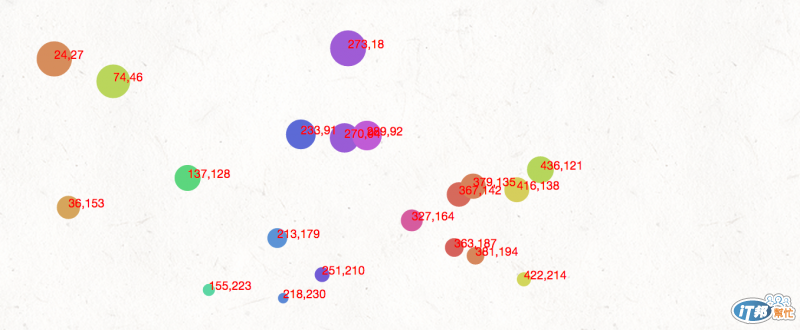#DAY 9
2

## Demo

var dataset = [[5, 20], [480, 90], [250, 50]]var w = 600;
var h = 250;

var dataset = [];
for (var i=0; i < 20; i++){
var Num1 = 5 + Math.floor(Math.random() * 480); //產生第一個數值
var Num2 = 5 + Math.floor(Math.random() * 230); //產生第二個數值
dataset.push([Num1, Num2]); //兩個合併成一個陣列
};
console.log(dataset); //檢查看看有沒有怪怪的

var svg = d3.select('.demo').append('svg').attr({'width': w,'height': h})
//產生一個SVG

svg.selectAll('circle').data(dataset).enter() //記得喔 data(dataset).enter() 把資料放入
.append('circle')
.attr({
'cx': function(d){return d + 10}, //定義圓心的x，在第一個值
'cy': function(d){return d + 10}, //定義圓心的y，在第二個值
'r': function(d){return Math.sqrt(h - d)}, //圓心的半徑，第二個值開平方
'fill': function(d){return d3.hsl(d % 360, .6, .6 );}
//介紹一個顏色的function hsl，可以將顏色算出後轉成色碼
//格式 (360色相, 1彩度, 1明度)
});

svg.selectAll('text').data(dataset).enter() //補上資料數值
.append('text')
.text(function(d){ return d+ ',' + d}) //將值寫到SVG上
.attr({
'x': function(d){return xScale(d)}, //和上面相同，算出X、Y的位置
'y': function(d){return yScale(d)},
'fill': 'red', //文字填滿為紅色
'font-size': '10px' //Fill、font-size也可以用CSS寫喔～
});

SVG 與 D3.js30